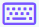Easy
Medium
Hard

## Partial derivative calculator with steps

Partial derivative calculator is used to calculate the derivatives of the functions w.r.t several variables with steps. This partial derivative solver differentiates the given constant, linear, or polynomial functions multiple times. It is a subtype of the derivative calculator.

## What is a partial derivative?

“In differential calculus, the differentiation of a function of multivariable w.r.t change in just one of its variables is known as a partial derivative.”

The equations of partial derivatives can be written as:

•    $$\frac{\partial }{\partial x}\left(f\left(x,y,z\right)\right)$$

The above equation is used for a multivariable function to calculate the partial differential w.r.t “x”.

•    $$\frac{\partial }{\partial y}\left(f\left(x,y,z\right)\right)$$

This equation used,  if a function has to be solved w.r.t “y”.

•    $$\frac{\partial }{\partial z}\left(f\left(x,y,z\right)\right)$$

This equation is used when the partial derivative of a function has to be solved w.r.t “z

Use our partial derivative calculator xyz for getting the results of multivariable problems.

## How to use this first partial derivative calculator?

To use this first partial differentiation calculator, follow the steps below.

• Input the multivariable function like f(x, y, z).
• Choose one variable from x, y, and z while other variables remain constant.
• Use the keypad iconto enter math keys i.e., +, x, ^, etc.
• Hit the calculate button to get the result of the given input function.
• If you want to calculate another problem hit the reset button next to the calculate button.

## How to evaluate the problems of partial derivatives?

Following are two examples of the 3 variables function evaluated by our multivariable derivative calculator.

Example 1

Find the partial derivative of 3xyz w.r.t “x”.

Solution

Step 1: Write the function with the partial differentiation notation.

$$\frac{\partial }{\partial x}\left(3xyz\right)$$

Step 2: Now calculate the partial derivative of 3xyz w.r.t “x” while y & z remains constant.

$$\frac{\partial }{\partial x}\left(3xyz\right)=3yz\frac{\partial }{\partial x}\left(x\right)$$

$$\frac{\partial }{\partial x}\left(3xyz\right)=3yz\left(1\right)$$

$$\frac{\partial }{\partial x}\left(3xyz\right)=3yz$$

Step 3: Similarly, the partial derivative of 3xyz w.r.t y & z are:

$$\frac{\partial }{\partial y}\left(3xyz\right)=3xz$$

$$\frac{\partial }{\partial z}\left(3xyz\right)=3xy$$

Example 2

Find the partial derivative of $$3x^2y+4xyz-9xy$$ w.r.t “y”.

Solution

Step 1: Write the given function along with the partial derivative notation.

$$\frac{\partial }{\partial y}\left(3x^2y+4xyz-9xy\right)$$

Step 2: Now apply the notation separately.

$$\frac{\partial }{\partial y}\left(3x^2y+4xyz-9xy\right)=\frac{\partial }{\partial y}\left(3x^2y\right)+\frac{\partial }{\partial y}\left(4xyz\right)-\frac{\partial }{\partial y}\left(9xy\right)$$

Step 3: Now calculate the partial derivative of $$3x^2y+4xyz-9xy$$ w.r.t “y” while x & z remains constant.

$$\frac{\partial }{\partial y}\left(3x^2y+4xyz-9xy\right)=3x^2\frac{\partial }{\partial y}\left(y\right)+4xz\frac{\partial }{\partial y}\left(y\right)-9x\frac{\partial }{\partial y}\left(y\right)$$

$$\frac{\partial }{\partial y}\left(3x^2y+4xyz-9xy\right)=3x^2\left(1\right)+4xz\left(1\right)-9x\left(1\right)$$

$$\frac{\partial }{\partial y}\left(3x^2y+4xyz-9xy\right)=3x^2+4xz-9x$$

Step 4: Similarly, the partial derivative of given function w.r.t x & z are:

$$\frac{\partial }{\partial x}\left(3x^2y+4xyz-9xy\right)=6xy+4yz-9y$$

$$\frac{\partial }{\partial z}\left(3x^2y+4xyz-9xy\right)=4xy$$

## Table of partial derivatives of functions

Following are questions and answers of partial derivatives solved by this partial derivatives calculator.

 Questions Answers Partial derivative of xy w.r.t x y Partial derivative of xy w.r.t y x Partial derivative of xy w.r.t z 0 Partial derivative of e^xy w.r.t x $$ye^{xy}$$ Partial derivative of e^xyz w.r.t x $$yze^{xyz}$$ Partial derivative of sqrt(xy) w.r.t x $$\frac{y}{2\sqrt{xy}\:}$$

### References

Use android or iOS app of our limit calculator on your mobile